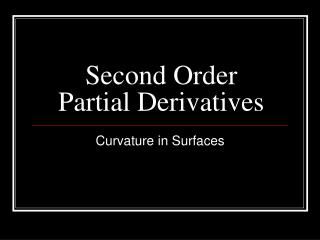DownloadDownload PresentationSecond Order Partial Derivatives

# Second Order Partial Derivatives

Download Presentation## Second Order Partial Derivatives

- - - - - - - - - - - - - - - - - - - - - - - - - - - E N D - - - - - - - - - - - - - - - - - - - - - - - - - - -
##### Presentation Transcript

1. Second Order Partial Derivatives Curvature in Surfaces

2. We know that fx(P) measures the slope of the graph of f at the point P in the positive x direction. So fxx(P) measures the rate at which this slope changes when y is held constant. That is, it measures the concavity of the graph along the x-cross section through P. The “un-mixed” partials: fxx and fyy Likewise, fyy(P) measures the concavity of the graph along the y-cross section through P.

3. The “un-mixed” partials: fxx and fyy fxx(P) is Positive Negative Zero Example 1 What is the concavity of the cross section along the black dotted line?

4. The “un-mixed” partials: fxx and fyy fyy(P) is Positive Negative Zero Example 1 What is the concavity of the cross section along the black dotted line?

5. The “un-mixed” partials: fxx and fyy Example 2 fxx(Q) is Positive Negative Zero What is the concavity of the cross section along the black dotted line?

6. The “un-mixed” partials: fxx and fyy fyy(Q) is Positive Negative Zero Example 2 What is the concavity of the cross section along the black dotted line?

7. The “un-mixed” partials: fxx and fyy fxx(R) is Positive Negative Zero Example 3 What is the concavity of the cross section along the black dotted line?

8. The “un-mixed” partials: fxx and fyy fxx(R) is Positive Negative Zero Example 3 What is the concavity of the cross section along the black dotted line?

9. The “un-mixed” partials: fxx and fyy Note: The surface is concave up in the x-direction and concave down in the y-direction; thus it makes no sense to talk about the concavity of the surface at R. A discussion of concavity for the surface requires that we specify a direction. Example 3

10. The mixed partials: fxy and fyx fxy(P) is Positive Negative Zero Example 1 What happens to the slope in the x direction as we increase the value of y right around P? Does it increase, decrease, or stay the same?

11. The mixed partials: fxy and fyx fyx(P) is Positive Negative Zero Example 1 What happens to the slope in the y direction as we increase the value of x right around P? Does it increase, decrease, or stay the same?

12. The mixed partials: fxy and fyx Example 2 fxy(Q) is Positive Negative Zero What happens to the slope in the x direction as we increase the value of y right around Q? Does it increase, decrease, or stay the same?

13. The “un-mixed” partials: fxy and fyx fyx(Q) is Positive Negative Zero Example 2 What happens to the slope in the y direction as we increase the value of x right around Q? Does it increase, decrease, or stay the same?

14. The mixed partials: fyx and fxy fxy(R) is Positive Negative Zero Example 3 What happens to the slope in the x direction as we increase the value of y right around R? Does it increase, decrease, or stay the same?

15. The mixed partials: fyx and fxy fyx(R) is Positive Negative Zero Example 3 ? What happens to the slope in the y direction as we increase the value of x right around R? Does it increase, decrease, or stay the same?

16. The mixed partials: fyx and fxy fyx(R) is Positive Negative Zero Example 3 What happens to the slope in the y direction as we increase the value of x right around R? Does it increase, decrease, or stay the same?

17. Sometimes it is easier to tell. . . fyx(R) is Positive Negative Zero Example 4 W What happens to the slope in the y direction as we increase the value of x right around W? Does it increase, decrease, or stay the same?

18. To see this better. . . What happens to the slope in the y direction as we increase the value of x right around W? Does it increase, decrease, or stay the same? The “cross” slopes go from Positive to negative Negative to positive Stay the same Example 4 W

19. To see this better. . . • fyx(R) is • Positive • Negative • Zero Example 4 W